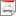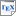[BKN99] C. Baier, M. Kwiatkowska and G. Norman. Computing Probability Lower and Upper Bounds for LTL Formulae over Sequential and Concurrent Markov Chains. In C. Baier and M. Huth and M. Kwiatkowska and M. Ryan (editors), Proc. 1st Probabilistic Methods in Verification Workshop (PROBMIV'98), volume 22 of Electronic Notes in Theoretical Computer Science, Elsevier Science. June 1999. [ps.gz] [pdf] [bib] Downloads:ps.gz (109 KB)pdf (246 KB)bib Notes: ENTCS is available at www.sciencedirect.com/science/journal/15710661. Links: [Google] [Google Scholar] [CiteSeer] Abstract. Probabilistic verification of concurrent probabilistic systems against linear time specifications is known to be expensive in terms of time and space: time is double exponential in the size of the formula and polynomial in the size of the state space, and space complexity is single exponential. This paper proposes to compute for a linear time formula only a lower and upper bound on the probability measure of the set of paths satisfying it, instead of calculating the exact probability. This yields a coarser estimate, namely an interval of values in [0,1] which contains the actual probability, but the calculation is simpler and more efficient (time is single exponential and space complexity is linear), and could thus be useful as an initial check in a model checking tool.